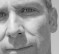(So far, 260 people got it right out of 284 for a success rate of 91%)

In C, what's the fastest way (execution wise) to divide an unsigned integer by 4?

(Assumption: the number will be divisible by 4 evenly).

Pick one:
NewNumber = Number / 4; (FPU disabled)
NewNumber = Number / 4; (FPU enabled)
NewNumber = Number >> 2;
#define DIVIDE(Number) (Number/4)

[ - ]
Comment by October 19, 2022I think there should have been a 5th possible answer, "It depends on the compiler". I would have thought a decent modern compiler would produce the same code for all of them. As already noted older (or poorer) compilers may code the C literally and actually do a divide.

[ - ]
Comment by October 21, 2022^^ This is the answer, but I would state as your second sentence: "I would have thought a decent modern compiler would produce the same code for all of them."

It does, even ancient versions of gcc with -O0. Why any compiler writer in their right mind would use a divide instruction rather than a shift right is beyond me.

Try this in Compiler Explorer (https://www.godbolt.org) :

#include <stdint.h>

uint32_t quiz1(uint32_t n)
{
return n/4;
}

uint32_t quiz2(uint32_t n)
{
return n>>2;
}

uint32_t quiz3(uint32_t n)
{
#define DIVIDE(Number) (Number/4)
return DIVIDE(n);
}
[ - ]
Comment by October 19, 2022In C, what's the fastest way (execution wise) to divide ANY number by 4 in C?

but you can't use >> for floating point numbers. This example gives you a compile error:

double ff;
double fff;
ff=4.0;
fff = ff >> 2;

>> is an old trick for integers. Also used when you can express your desired scale factor as a fraction where your fraction has a denominator that is a power of 2, iii = ii * n / (2^n). Just a multiply and a shift.

[ - ]
Comment by October 19, 2022Great catch!  We've modified the question from 'any' to 'unsigned integer'.  Thanks!

[ - ]
Comment by October 19, 2022Your bit shifting is incorrect in the article above.

I have corrected it for you below.

Divide by 2:Number >> 1

Divide by 4:Number >> 2

Divide by 8:Number >> 3

Divide by 16: Number >> 4

[ - ]
Comment by October 19, 2022Corrected, thanks!

[ - ]
Comment by November 16, 2022Hello,

I agree with the fact that right-shifting is faster than dividing by 4. But, as you mentioned in your last sentence, every decent compiler would be able to replace multiplications and divisions by powers of 2 by bit shifts. In 2022, I really hope that everyone has this kind of tool!

And using a macro would speed up the execution more, if Number can be known at compile time. In this case, the division would be immediately replaced by its result by the preprocessor, and so the instruction would be even faster. But this is not very readable... So in a professional context, I would prefer the option 2. KISS: Keep It Simple and Smart

To post reply to a comment, click on the 'reply' button attached to each comment. To post a new comment (not a reply to a comment) check out the 'Write a Comment' tab at the top of the comments.